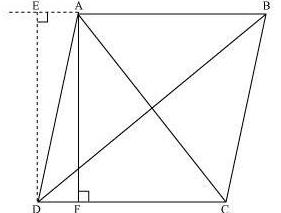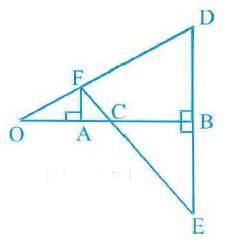## Pages

### NCERT Maths 10th triangle solved optional questions

Prove that the sum of the squares of the diagonals of parallelogram is equal to the sum of the squares of its sides.Given that ABCD be a parallelogram.

Construction : We have to Draw AF and DE perpendicular on side DC and on extended side AB to use Pythagoras theorem

In ΔDEA, DE2 + EA2 = DA2 … (i)

Similarly, In ΔDEB,

DE2 + EB2 = DB2

DE2 + (EA + AB)2 = DB2 ( EB =EA + AB)

DE2 + EA2 + AB2 + 2EA × AB == DB2

(DE2 + EA2) + AB2 + 2EA × AB = DB2

DA2 + AB2 + 2EA × AB = DB2 … (ii)( Using -----1)

In ΔAFC, AC2 = AF2 + FC2

= AF2 + (DC − FD)2

= AF2 + DC2 + FD2 − 2DC × FD

= (AF2 + FD2) + DC2 − 2DC × FD

=AC2 = AD2 + DC2 − 2DC × FD ( using AD2 = AF2 + FD2)… (iii)

Now,given that ABCD is a parallelogram, AB = CD … (iv) And, BC = AD … (v)

Look In ΔDEA and ΔADF,

∠DEA = ∠AFD (Both 90°)

∠EAD = ∠ADF (EA || DF -Alternate angles )

AD = AD (Common)

∴ ΔEAD cong. ΔFDA (AAS congruence criterion)

⇒ EA = DF … (vi)
Adding equations (i) and (iii), we obtain

DA2 + AB2 + 2EA × AB + AD2 + DC2 − 2DC × FD = DB2 + AC2

DA2 + AB2 + AD2 + DC2 + 2EA × AB − 2DC × FD = DB2 + AC2

BC2 + AB2 + AD2 + DC2 + 2EA × AB − 2AB × EA = DB2 + AC2
[Using equations (iv) and (vi)]

AB2 + BC2 + CD2 + DA2 = AC2 + BD2

Q. In Fig. is the perpendicular bisector of the line segment DE, FA perpendiculat OB and F E intersects OB at the point C. Prove that 1/ OA + 1/OB = 1/OCInΔ AOF and ΔBOD

< O = <O (Same angle) and <A = <B (each 90°)

Therefore, ΔAOF ~ ΔBOD (AA similarity)

So,OA/OB = FA/BD ------(1) (B.P.T)

Also, in ΔFAC and ΔEBC,

< A = <B (Each 90°)

< FCA = <ECB (Vertically opposite angles).

ΔFAC ~ ΔEBC (AA similarity).

So, FA/EB = AC/BC------(2)

But EB = DB (B is mid-point of DE)

Therefore,using (1) and (2)

AC/BC = OA/OB

OC–OA/ OB–OC = OA /OB

or OB . OC – OA . OB = OA . OB – OA . OC

or OB . OC + OA . OC = 2 OA . OB
or (OB + OA). OC = 2 OA . OB

1/ OA + 1/OB = 1/OC [ Dividing both the sides by OA . OB . OC]

Q. In the given figure, D is a point on side BC of ΔABC such that BD/ CD = AB/AC. Prove that AD is the bisector of ∠BAC.Proof: Construction needed : Extend BA to P such that AP = AC then join PC

Given that BD/ CD = AB/AC but AP = AC

BD/ CD = AB/AP
AD || PC ( by using the converse of B.P.T.)

⇒ ∠BAD = ∠APC (Corresponding angles) … (1)

also, ∠DAC = ∠ACP (Alternate interior angles) … (2)But ∠APC = ∠ACP … (3) ( as AP = AC)

Using (1), (2), and (3), we obtain

∠BAD = ∠APC

⇒ AD is the bisector of the angle BAC.

Q.Nazima is fly fishing in a stream. The tip of her fishing rod is 1.8 m above the surface of the water and the fly at the end of the string rests on the water 3.6 m away and 2.4 m from a point directly under the tip of the rod. Assuming that her string (from the tip of her rod to the fly) is taut, ho much string does she have out ? If she pulls in the string at the rate of 5 cm per second, what will be the horizontal distance of the fly from her after 12 seconds?

Let AB and BC be the height of the tip of the fishing rod from the water surface and the horizontal distance of the fly from the tip of the fishing rod. We get a triangle ABC
she pulls in the string at the rate of 5 cm per second for 12 seconds = 12 × 5 = 60 cm = 0.6 m

In Δ ABC. AC2 = AB2 + BC2 AB2 = (1.8 m)2 + (2.4 m)2

Þ AB = 3 m
String pulled in 12 seconds to point D by pulling 0.6 m
but AC is the length of the original string.
Now AD is the length of the new string = 3.00 − 0.6 = 2.4 m
In ΔADB, AB2 + BD2 = AD
(1.8 m)2 + BD2 = (2.4 m)2
BD = 1.587 m
So, the horizontal distance of the fly from her after 12 seconds = (1.587 + 1.2) m
= 2.79 m